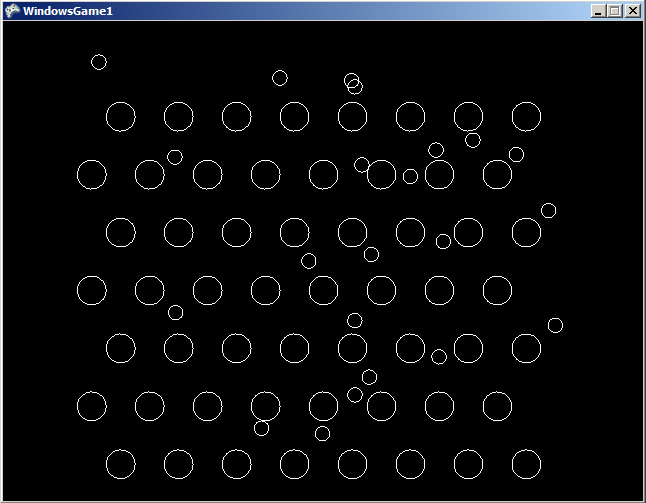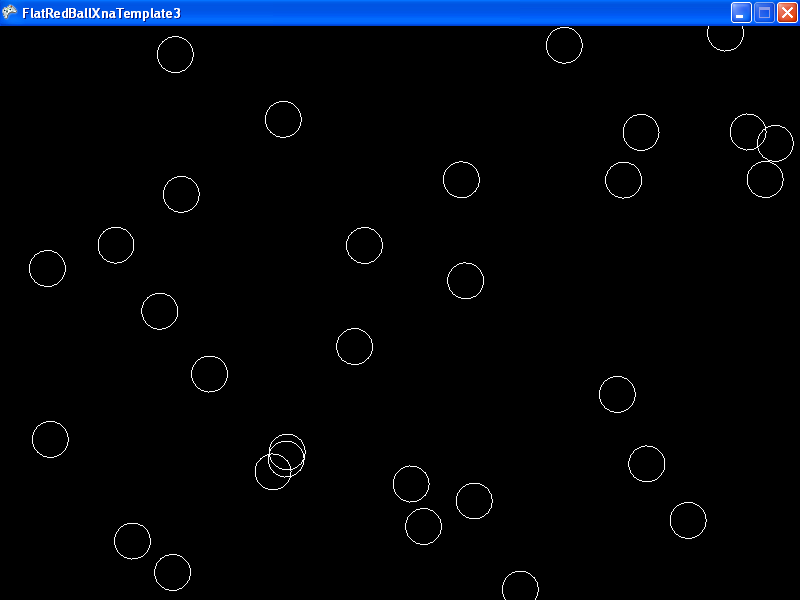# FlatRedBall.Math.Geometry.Polygon.CollideAgainstBounce

## Introduction

The CollideAgainstBounce is a collision method which performs the following:

• Returns true if a collision has occurred.
• Repositions the calling Circle and/or the argument object depending on the argument masses.
• Changes the calling Shape’s Velocity and/or the argument object’s Velocity depending on the argument masses.

Note: All collision methods, including CollideAgainstBounce, are methods common to all Shapes. If you came here through a link on a page beside the Circle (such as Polygon), don’t worry, the code for all shapes is identical.

## Method Signature

The signature for CollideAgainstBounce is as follows:

```bool CollideAgainstBounce(Circle circle, float thisMass, float otherMass, float elasticity)
bool CollideAgainstBounce(Polygon polygon, float thisMass, float otherMass, float elasticity)
bool CollideAgainstBounce(AxisAlignedRectangle axisAlignedRectangle, float thisMass, float otherMass, float elasticity)
```

Arguments:

• Circle circle (or other shape) – the shape to collide against. This method supports other shapes as well, and if you find a shape that you’d like to collide against which is not supported, please request this feature in the forums.
• float thisMass – the mass of this shape. This does not have to be an absolute mass since it will only be used relative to the otherMass argument.
• float otherMass – the mass of the argument shape. Just like thisMass, otherMass does not have to be absolute. It will be compared against thisMass.
• float elasticity – The amount of bounce that will result. A value of 1 results in all momentum being preserved through the collision. A value of 0 results in no momentum being preserved. Negative values should not be used. Values greater than 1 introduce extra momentum. Values greater than 1 can be used to simulate “bouncing” against a wound-up spring, or to create false physics. For more information on the elasticity  variable, see the Elasticity Examples section below.## Example Code

The following code creates a Plinko board.

```using FlatRedBall.Input;
using FlatRedBall.Math.Geometry;
using Microsoft.Xna.Framework.Input;
```
Add the following at class scope:
```PositionedObjectList<Circle> mPegs = new PositionedObjectList<Circle>();
PositionedObjectList<Circle> mFallingPieces = new PositionedObjectList<Circle>();
```
Add the following in Initialize after initializing FlatRedBall:
``` for (int i = 0; i < 8; i++)
{
for (int j = 0; j < 7; j++)
{
circle.X = -16 + 4 * i;
circle.Y = -14 + 4 * j;

if (j % 2 == 0)
circle.X += 2;

}
}
```
``` if (InputManager.Keyboard.KeyPushed(Keys.Space))
{
circle.YAcceleration = -5;
circle.Y = 16;

circle.X = -16 + (float)FlatRedBallServices.Random.NextDouble() * 32;

}

foreach (Circle fallingPiece in mFallingPieces)
{
foreach (Circle peg in mPegs)
{
// The second argument (0) is the mass of the falling piece relative to the peg.
// The third argument (1) is the mass of the peg relative to the falling piece.
// The 0,1 combination makes the peg of infinite mass - it will never move.
// The last argument (.75) makes the collision slightly inelastic so it
// looks a little more realistic.
fallingPiece.CollideAgainstBounce(peg, 0, 1, .75f);
}
}
```## Reacting to CollideAgainstBounce

Since CollideAgainstBounce returns a bool, your code can use the return value to modify your game when a collision occurs:

```bool didCollisionOccur = circle.CollideAgainstBounce(otherCircle, 0, 1, 1);
if(didCollisionOccur)
{
// Here you can do whatever you want, like play a sound effect, add points, or even modify the velocity
// of either of the objects
}
```

## Bouncing and Velocity

The Collision tutorial mentions:

```"For bouncing to behave properly, we have to make sure that we're not controlling the involved Shapes' position or velocity"
```

Let’s consider why this is the case. Imagine a situation where a shape (or parent of a shape) is moving toward the right. This is being done with the following code:

```someShape.XVelocity = 1;
```

If this code is called every frame, that means that the XVelocity will be set to 1 every frame, regardless of what it was before. Imagine that “someShape” performs bounce collision against a wall. When collision occurs, the shape’s XVelocity will get inverted (that is, set to -1) so that it moves to the left. However, if Velocity is set to 1 every frame, than the -1 would get changed back to 1. In other words, the setting of velocity every frame would cancel out the velocity change from CollideAgainstBounce.

Let’s look at another example: one where an object is moved with the mouse. In this example, the mouse is simply setting the position of an object:

```someShape.X = InputManager.Mouse.WorldXAt(0);
someShape.Y = InputManager.Mouse.WorldYAt(0);
```

In this case, someShape will have a velocity of 0 (assuming there is no other code that sets its velocity. Therefore, even though someShape appears to be moving smoothly with the mouse, if another object bounces against it, it may not bounce at all.

### Code Example

The following example shows a problem with using CollideAgainstBounce and Mouse control and how it can be corrected.

```using FlatRedBall;
using FlatRedBall.Input;
using FlatRedBall.Math;
using FlatRedBall.Math.Geometry;
```
Add the following at class scope:
```PositionedObjectList<Circle> mCircles = new PositionedObjectList<Circle>();
Circle mControlledCircle;
```
Add the following in Initialize after initializing FlatRedball:
``` mControlledCircle = ShapeManager.AddCircle();

for (int i = 0; i < 30; i++)
{
SpriteManager.Camera.PositionRandomlyInView(circle, 40, 40);
}
```
``` // true will result in no bouncing
// false will result in bouncing (probably expected behavior)

{
mControlledCircle.X = InputManager.Mouse.WorldXAt(0);
mControlledCircle.Y = InputManager.Mouse.WorldYAt(0);
}
else
{
// Increasing this reduces lag, but increases potential jittery
// velocity values.
const float coefficient = 20;

mControlledCircle.XVelocity = coefficient *
(InputManager.Mouse.WorldXAt(0) - mControlledCircle.X);
mControlledCircle.YVelocity = coefficient *
(InputManager.Mouse.WorldYAt(0) - mControlledCircle.Y);
}
foreach (Circle circle in mCircles)
{
// Make this mass 1, the other mass 0 so that this is
// immovable and it will bounce and push all other objects.
mControlledCircle.CollideAgainstBounce(circle, 1, 0, 1);
}
```## CollideAgainstBounce for platformers

The CollideAgainstBounce method is effective for performing bouncing physics, but it can also be used in situations where you’d like collision to reset velocity, such as in platformers.

It’s very common to have acceleration in platformers, and the easiest way to do this is to set the player Entity to have a negative YAcceleration. However, if the player collides with the ground using CollideAgainstMove, the YAcceleration will continue to accumulate the YVelocity value. Eventually this value will build up to be so large that the player will fall through the level. Even if this doesn’t occur, the player will show weird behavior if it walks off of a ledge.

To solve this, you can simply use CollideAgainstBounce instead of CollideAgainstMove. An elasticity of 0 will result in the same behavior as CollideAgainstMove, but the velocity will be modified according to the velocity to solve accumulation errors. You can try this in the demo above by setting the elasticity argument to 0.